# Formulating Algorithms: Sentinel-Controlled Repetition

### Formulating Algorithms Sentinel Controlled Repetition

Let us generalize Section 5.8's class-average problem. Consider the following problem:

Develop a class-averaging application that processes grades for an arbitrary number of
students each time it is run.

In the previous class-average example, the problem statement specified the number of students, so the number of grades (10) was known in advance. In this example, no indication is given of how many grades the user will enter during the application's execution. The application must process an arbitrary number of grades. How can it determine when to stop the input of grades? How will it know when to calculate and print the class average?

One way to solve this problem is to use a special value called a sentinel value (also called a signal value, a dummy value or a flag value) to indicate "end of data entry." This is called sentinel-controlled repetition. The user enters grades until all legitimate grades have been entered. The user then types the sentinel value to indicate that no more grades will be entered. Sentinel-controlled repetition is often called indefinite repetition because the number of repetitions is not known by the application before the loop begins executing.

Clearly, a sentinel value must be chosen that cannot be confused with an acceptable input value. Grades on a quiz are nonnegative integers, so 1 is an acceptable sentinel value for this problem. Thus, a run of the class-average application might process a stream of inputs such as 95, 96, 75, 74, 89 and 1. The application would then compute and print the class average for the grades 95, 96, 75, 74 and 89. Since 1 is the sentinel value, it should not enter into the averaging calculation.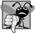## Common Programming Error 5 6

Choosing a sentinel value that is also a legitimate data value is a logic error.

Developing the Pseudocode Algorithm with Top-Down, Stepwise Refinement: The Top and First Refinement

We approach the class-average application with a technique called top-down, stepwise refinement, which is essential to the development of well-structured applications. We begin with a pseudocode representation of the topa single statement that conveys the overall function of the application:

determine the class average for the quiz

The top is, in effect, a complete representation of an application. Unfortunately, the top rarely conveys sufficient detail from which to write a C# application. So we now begin the refinement process. We divide the top into a series of smaller tasks and list these in the order in which they will be performed. This results in the following first refinement:

initialize variables
input, sum and count the quiz grades
calculate and print the class average

This refinement uses only the sequence structurethe steps listed should execute in order, one after the other.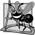## Software Engineering Observation 5 2

Each refinement, as well as the top itself, is a complete specification of the algorithmonly the level of detail varies.## Software Engineering Observation 5 3

Many applications can be divided logically into three phases: an initialization phase that initializes the variables; a processing phase that inputs data values and adjusts application variables (e.g., counters and totals) accordingly; and a termination phase that calculates and outputs the final results.

Proceeding to the Second Refinement

The preceding Software Engineering Observation is often all you need for the first refinement in the top-down process. To proceed to the next level of refinement, the second refinement, we specify individual variables. In this example, we need a running total of the numbers, a count of how many numbers have been processed, a variable to receive the value of each grade as it is input by the user and a variable to hold the calculated average. The pseudocode statement

initialize variables

can be refined as follows:

initialize total to zero
initialize counter to zero

Only the variables total and counter need to be initialized before they are used. The variables average and grade (for the calculated average and the user input, respectively) need not be initialized, because their values will be replaced as they are calculated or input.

The pseudocode statement

input, sum and count the quiz grades

requires a repetition structure (i.e., a loop) that successively inputs each grade. We do not know in advance how many grades are to be processed, so we will use sentinel-controlled repetition. The user enters grades one at a time. After entering the last grade, the user enters the sentinel value. The application tests for the sentinel value after each grade is input and terminates the loop when the user enters the sentinel value. The second refinement of the preceding pseudocode statement is then

prompt the user to enter the first grade
input the first grade (possibly the sentinel)

while the user has not yet entered the sentinel
prompt the user to enter the next grade
input the next grade (possibly the sentinel)

In pseudocode, we do not use braces around the statements that form the body of the while structure. We simply indent the statements under the while to show that they belong to the while. Again, pseudocode is only an informal application-development aid.

The pseudocode statement

calculate and print the class average

can be refined as follows:

if the counter is not equal to zero
set the average to the total divided by the counter
print the average
else

We are careful here to test for the possibility of division by zeroa logic error that, if undetected, would cause the application to fail or produce invalid output. The complete second refinement of the pseudocode for the class-average problem is shown in Fig. 5.8.

Figure 5.8. Class-average problem pseudocode algorithm with sentinel-controlled repetition.

 ``` 1 initialize total to zero 2 initialize counter to zero 3 4 prompt the user to enter the first grade 5 input the first grade (possibly the sentinel) 6 7 while the user has not yet entered the sentinel 8 add this grade into the running total 9 add one to the grade counter 10 prompt the user to enter the next grade 11 input the next grade (possibly the sentinel) 12 13 if the counter is not equal to zero 14 set the average to the total divided by the counter 15 print the average 16 else 17 print "No grades were entered" ```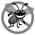## Error Prevention Tip 5 2

When performing division by an expression whose value could be zero, explicitly test for this possibility and handle it appropriately in your application (e.g., by printing an error message) rather than allowing the error to occur.

In Fig. 5.5 and Fig. 5.8, we included some completely blank lines and indentation in the pseudocode to make it more readable. The blank lines separate the pseudocode algorithms into their various phases and set off control statements, and the indentation emphasizes the bodies of the control statements.

The pseudocode algorithm in Fig. 5.8 solves the more general class-averaging problem. This algorithm was developed after only two refinements. Sometimes more refinements are necessary.## Software Engineering Observation 5 4

Terminate the top-down, stepwise refinement process when you have specified the pseudocode algorithm in sufficient detail for you to convert the pseudocode to C#. Normally, implementing the C# application is then straightforward.## Software Engineering Observation 5 5

Some experienced programmers write applications without ever using application-development tools like pseudocode. They feel that their ultimate goal is to solve the problem on a computer and that writing pseudocode merely delays the production of final outputs. Although this method may work for simple and familiar problems, it can lead to serious errors and delays in large, complex projects.

Implementing Sentinel-Controlled Repetition in Class GradeBook

Figure 5.9 shows the C# class GradeBook containing method DetermineClassAverage that implements the pseudocode algorithm of Fig. 5.8. Although each grade is an integer, the averaging calculation is likely to produce a number with a decimal pointin other words, a real number or floating-point number. The type int cannot represent such a number, so this class uses type double to do so.

Figure 5.9. GradeBook class that solves the class-average problem using sentinel-controlled repetition.

(This item is displayed on pages 192 - 193 in the print version)

 ``` 1 // Fig. 5.9: GradeBook.cs 2 // GradeBook class that solves class-average problem using 3 // sentinel-controlled repetition. 4 using System; 5 6 public class GradeBook 7 { 8 private string courseName; // name of course this GradeBook represents 9 10 // constructor initializes courseName 11 public GradeBook( string name ) 12 { 13 CourseName = name; // initialize courseName by using property 14 } // end constructor 15 16 // property to get and set the course name 17 public string CourseName 18 { 19 get 20 { 21 return courseName; 22 } // end get 23 set 24 { 25 courseName = value; // set should validate 26 } // end set 27 } // end property CourseName 28 29 // display a welcome message to the GradeBook user 30 public void DisplayMessage() 31 { 32 Console.WriteLine( "Welcome to the grade book for {0}! ", 33 CourseName ); 34 } // end method DisplayMessage 35 36 // determine the average of an arbitrary number of grades 37 public void DetermineClassAverage() 38 { 39 int total; // sum of grades 40 int gradeCounter; // number of grades entered 41 int grade; // grade value 42 double average; // number with decimal point for average 43 44 // initialization phase 45 total = 0; // initialize total 46 gradeCounter = 0; // initialize loop counter 47 48 // processing phase 49 // prompt for input and read grade from user 50 Console.Write( "Enter grade or -1 to quit: " ); 51 grade = Convert.ToInt32( Console.ReadLine() ); ``` 52 53 // loop until sentinel value read from user 54 while ( grade != -1 ) 55 { 56 total = total + grade; // add grade to total 57 gradeCounter = gradeCounter + 1; // increment counter 58 59 // prompt for input and read next grade from user 60 Console.Write( "Enter grade or -1 to quit: " ); 61 grade = Convert.ToInt32( Console.ReadLine() ); 62 } // end while 63 64 // termination phase 65 // if user entered at least one grade... 66 if ( gradeCounter != 0 ) 67 { 68 // calculate average of all grades entered 69 average = ( double ) total / gradeCounter; 70 71 // display total and average (with two digits of precision) 72 Console.WriteLine( " Total of the {0} grades entered is {1}", 73 gradeCounter, total ); 74 Console.WriteLine( "Class average is {0:F2}", average ); 75 } // end if 76 else // no grades were entered, so output error message 77 Console.WriteLine( "No grades were entered" ); 78 } // end method DetermineClassAverage 79 } // end class GradeBook

In this example, we see that control statements may be stacked on top of one another (in sequence) just as a child stacks building blocks. The while statement (lines 5462) is followed in sequence by an if...else statement (lines 6677). Much of the code in this application is identical to the code in Fig. 5.6, so we concentrate on the new features and issues.

Line 42 declares double variable average. This variable allows us to store the calculated class average as a floating-point number. Line 46 initializes gradeCounter to 0, because no grades have been entered yet. Remember that this application uses sentinel-controlled repetition to input the grades from the user. To keep an accurate record of the number of grades entered, the application increments gradeCounter only when the user inputs a valid grade value.

Program Logic for Sentinel-Controlled Repetition vs. Counter-Controlled Repetition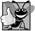## Good Programming Practice 5 7

In a sentinel-controlled loop, the prompts requesting data entry should explicitly remind the user of the sentinel value.

After the loop terminates, the if...else statement at lines 6677 executes. The condition at line 66 determines whether any grades were input. If none were input, the else part (lines 7677) of the if...else statement executes and displays the message "No grades were entered", and the method returns control to the calling method.

Notice the while statement's block in Fig. 5.9 (lines 5562). Without the braces, the loop would consider its body to be only the first statement, which adds the grade to the total. The last three statements in the block would fall outside the loop's body, causing the computer to interpret the code incorrectly as follows:

```while ( grade != -1 )

Console.Write( "Enter grade or -1 to quit: " );
```

The preceding code would cause an infinite loop in the application if the user did not enter the sentinel -1 at line 51 (before the while statement).## Error Prevention Tip 5 3

Omitting the braces that delimit a block can lead to logic errors, such as infinite loops. To prevent this problem, some programmers enclose the body of every control statement in braces even if the body contains only a single statement.

Explicitly and Implicitly Converting Between Simple Types

If at least one grade was entered, line 69 of Fig. 5.9 calculates the average of the grades. Recall from Fig. 5.6 that integer division yields an integer result. Even though variable average is declared as a double (line 42), the calculation

```average = total / gradeCounter;
```

loses the fractional part of the quotient before the result of the division is assigned to average. This occurs because total and gradeCounter are both integers, and integer division yields an integer result. To perform a floating-point calculation with integer values, we must temporarily treat these values as floating-point numbers for use in the calculation. C# provides the unary cast operator to accomplish this task. Line 69 uses the (double) cast operatora unary operatorto create a temporary floating-point copy of its operand total (which appears to the right of the operator). Using a cast operator in this manner is called explicit conversion. The value stored in total is still an integer.

The calculation now consists of a floating-point value (the temporary double version of total) divided by the integer gradeCounter. C# knows how to evaluate only arithmetic expressions in which the operands' types are identical. To ensure that the operands are of the same type, C# performs an operation called promotion (or implicit conversion) on selected operands. For example, in an expression containing values of the types int and double, the int values are promoted to double values for use in the expression. In this example, the value of gradeCounter is promoted to type double, then floating-point division is performed and the result of the calculation is assigned to average. As long as the (double) cast operator is applied to any variable in the calculation, the calculation will yield a double result. Later in this chapter, we discuss all the simple types. You will learn more about the promotion rules in Section 7.7.## Common Programming Error 5 7

The cast operator can be used to convert between simple numeric types, such as int and double, and between related reference types (as we discuss in Chapter 11, Polymorphism, Interfaces & Operator Overloading). Casting to the wrong type may cause compilation errors or runtime errors.

Cast operators are available for all simple types. (We'll discuss cast operators for reference types in Chapter 11.) The cast operator is formed by placing parentheses around the name of a type. This operator is a unary operator (i.e., an operator that takes only one operand). In Chapter 3, we studied the binary arithmetic operators. C# also supports unary versions of the plus (+) and minus () operators, so you can write expressions like +5 or -7. Cast operators associate from right to left and have the same precedence as other unary operators, such as unary + and unary -. This precedence is one level higher than that of the multiplicative operators *, / and %. (See the operator precedence chart in Appendix A.) We indicate the cast operator with the notation (type) in our precedence charts, to indicate that any type name can be used to form a cast operator.

Line 74 outputs the class average using Console's WriteLine method. In this example, we decided that we'd like to display the class average rounded to the nearest hundredth and output the average with exactly two digits to the right of the decimal point. The format specifier F in WriteLine's format item (line 74) indicates that variable average's value should be displayed as a real number. The number after the format specifier F represents the number of decimal places (in this case, 2) that should be output to the right of the decimal point in the floating-point numberalso known as the number's precision. Any floating point value output with F2 will be rounded to the hundredths positionfor example, 123.457 would be rounded to 123.46, and 27.333 would be rounded to 27.33. In this application, the three grades entered during the sample execution of class GradeBookTest (Fig. 5.10) total 263, which yields the average 87.66666.... The format item rounds the average to the hundredths position, and the average is displayed as 87.67.

Figure 5.10. Create GradeBook object and invoke its DetermineClassAverage method.

``` 1 // Fig. 5.10: GradeBookTest.cs
2 // Create GradeBook object and invoke its DetermineClassAverage method.
4 {
5 public static void Main( string[] args )
6 {
8 // pass course name to constructor
10 "CS101 Introduction to C# Programming" );
11
12 myGradeBook.DisplayMessage(); // display welcome message
14 } // end Main
15 } // end class GradeBookTest
```

 ```Welcome to the grade book for CS101 Introduction to C# Programming! Enter grade or -1 to quit: 96 Enter grade or -1 to quit: 88 Enter grade or -1 to quit: 79 Enter grade or -1 to quit: -1 Total of the 3 grades entered is 263 Class average is 87.67 ```

### Formulating Algorithms Nested Control Statements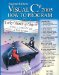Visual C# 2005 How to Program (2nd Edition)
ISBN: 0131525239
EAN: 2147483647
Year: 2004
Pages: 600